Anzeige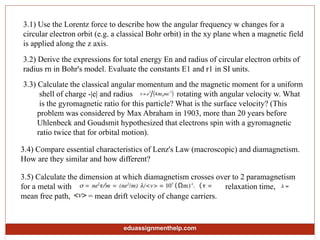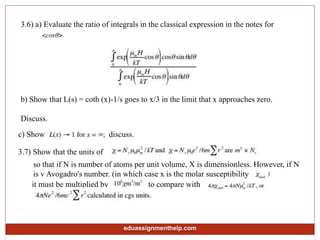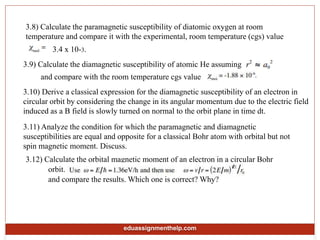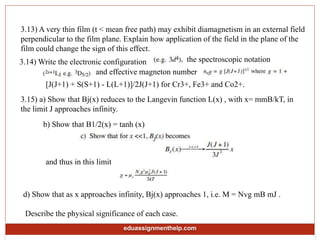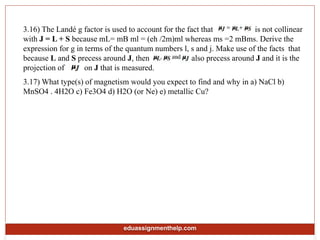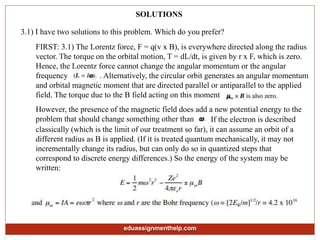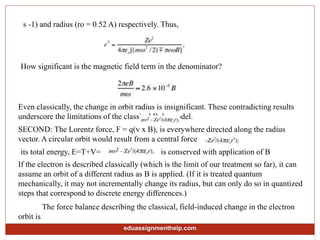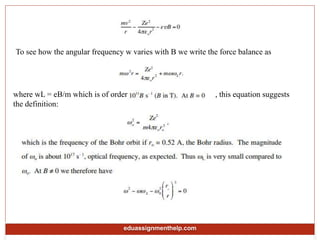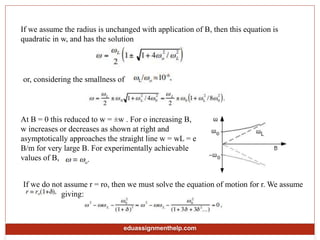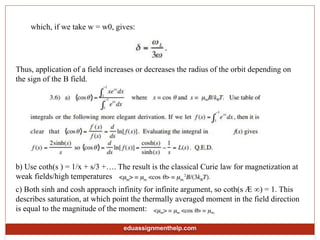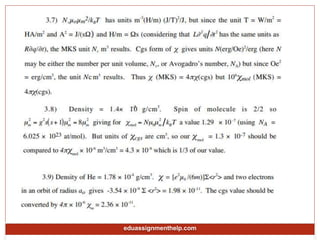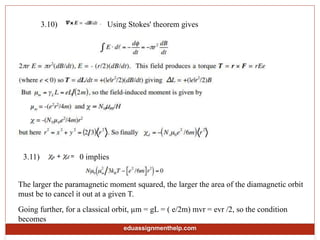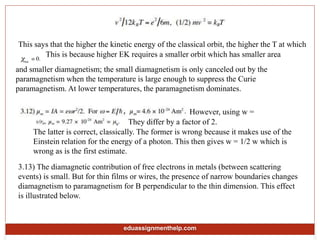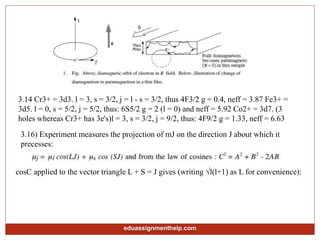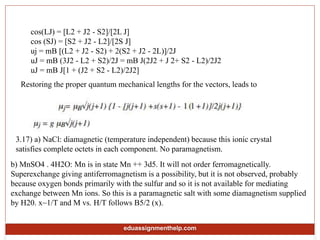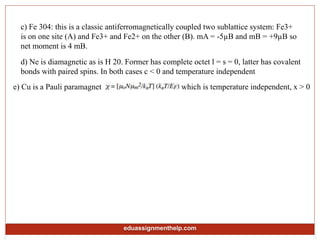1 von 17
Anzeige

### Magnetic Materials Assignment Help

1. Magnetic Materials Assignment Help For any help regarding Magnetic Materials Assignment Help Visit :- https://www.eduassignmenthelp.com/ Email :- info@eduassignmenthelp.com call us at :- +1 678 648 4277
2. 3.1) Use the Lorentz force to describe how the angular frequency w changes for a circular electron orbit (e.g. a classical Bohr orbit) in the xy plane when a magnetic field is applied along the z axis. 3.2) Derive the expressions for total energy En and radius of circular electron orbits of radius rn in Bohr's model. Evaluate the constants E1 and r1 in SI units. 3.3) Calculate the classical angular momentum and the magnetic moment for a uniform shell of charge -|e| and radius rotating with angular velocity w. What is the gyromagnetic ratio for this particle? What is the surface velocity? (This problem was considered by Max Abraham in 1903, more than 20 years before Uhlenbeck and Goudsmit hypothesized that electrons spin with a gyromagnetic ratio twice that for orbital motion). 3.4) Compare essential characteristics of Lenz's Law (macroscopic) and diamagnetism. How are they similar and how different? 3.5) Calculate the dimension at which diamagnetism crosses over to 2 paramagnetism for a metal with relaxation time, mean free path, = mean drift velocity of change carriers. eduassignmenthelp.com
3. 3.6) a) Evaluate the ratio of integrals in the classical expression in the notes for b) Show that L(s) = coth (x)-1/s goes to x/3 in the limit that x approaches zero. Discuss. c) Show ; discuss. 3.7) Show that the units of so that if N is number of atoms per unit volume, X is dimensionless. However, if N is v Avogadro's number. (in which case x is the molar susceptibility it must be multiplied by to compare with eduassignmenthelp.com
4. 3.8) Calculate the paramagnetic susceptibility of diatomic oxygen at room temperature and compare it with the experimental, room temperature (cgs) value 3.4 x 10-3. 3.9) Calculate the diamagnetic susceptibility of atomic He assuming and compare with the room temperature cgs value 3.10) Derive a classical expression for the diamagnetic susceptibility of an electron in circular orbit by considering the change in its angular momentum due to the electric field induced as a B field is slowly turned on normal to the orbit plane in time dt. 3.11) Analyze the condition for which the paramagnetic and diamagnetic susceptibilities are equal and opposite for a classical Bohr atom with orbital but not spin magnetic moment. Discuss. 3.12) Calculate the orbital magnetic moment of an electron in a circular Bohr orbit. and compare the results. Which one is correct? Why? eduassignmenthelp.com
5. 3.13) A very thin film (t < mean free path) may exhibit diamagnetism in an external field perpendicular to the film plane. Explain how application of the field in the plane of the film could change the sign of this effect. 3.14) Write the electronic configuration the spectroscopic notation and effective magneton number [J(J+1) + S(S+1) - L(L+1)]/2J(J+1) for Cr3+, Fe3+ and Co2+. 3.15) a) Show that Bj(x) reduces to the Langevin function L(x) , with x= mmB/kT, in the limit J approaches infinity. b) Show that B1/2(x) = tanh (x) and thus in this limit d) Show that as x approaches infinity, Bj(x) approaches 1, i.e. M = Nvg mB mJ . Describe the physical significance of each case. eduassignmenthelp.com
6. 3.16) The Landé g factor is used to account for the fact that is not collinear with J = L + S because mL= mB ml = (eh /2m)ml whereas ms =2 mBms. Derive the expression for g in terms of the quantum numbers l, s and j. Make use of the facts that because L and S precess around J, then also precess around J and it is the projection of on J that is measured. 3.17) What type(s) of magnetism would you expect to find and why in a) NaCl b) MnSO4 . 4H2O c) Fe3O4 d) H2O (or Ne) e) metallic Cu? eduassignmenthelp.com
7. SOLUTIONS 3.1) I have two solutions to this problem. Which do you prefer? FIRST: 3.1) The Lorentz force, F = q(v x B), is everywhere directed along the radius vector. The torque on the orbital motion, T = dL/dt, is given by r x F, which is zero. Hence, the Lorentz force cannot change the angular momentum or the angular frequency . Alternatively, the circular orbit generates an angular momentum and orbital magnetic moment that are directed parallel or antiparallel to the applied field. The torque due to the B field acting on this moment However, the presence of the magnetic field does add a new potential energy to the problem that should change something other than classically (which is the limit of our treatment so far), it can assume an orbit of a different radius as B is applied. (If it is treated quantum mechanically, it may not incrementally change its radius, but can only do so in quantized steps that correspond to discrete energy differences.) So the energy of the system may be written: If the electron is described eduassignmenthelp.com
8. s -1) and radius (ro = 0.52 A) respectively. Thus, How significant is the magnetic field term in the denominator? Even classically, the change in orbit radius is insignificant. These contradicting results underscore the limitations of the classical Bohr model. SECOND: The Lorentz force, F = q(v x B), is everywhere directed along the radius vector. A circular orbit would result from a central force its total energy, E=T+V= If the electron is described classically (which is the limit of our treatment so far), it can assume an orbit of a different radius as B is applied. (If it is treated quantum mechanically, it may not incrementally change its radius, but can only do so in quantized steps that correspond to discrete energy differences.) is conserved with application of B The force balance describing the classical, field-induced change in the electron orbit is eduassignmenthelp.com
9. To see how the angular frequency w varies with B we write the force balance as where wL = eB/m which is of order , this equation suggests the definition: eduassignmenthelp.com
10. If we assume the radius is unchanged with application of B, then this equation is quadratic in w, and has the solution or, considering the smallness of At B = 0 this reduced to w = ±w . For o increasing B, w increases or decreases as shown at right and asymptotically approaches the straight line w = wL = e B/m for very large B. For experimentally achievable values of B, If we do not assume r = ro, then we must solve the equation of motion for r. We assume giving: eduassignmenthelp.com
11. which, if we take w = w0, gives: Thus, application of a field increases or decreases the radius of the orbit depending on the sign of the B field. b) Use coth(s ) = 1/x + s/3 +…. The result is the classical Curie law for magnetization at weak fields/high temperatures c) Both sinh and cosh appraoch infinity for infinite argument, so coth(s Æ ∞) = 1. This describes saturation, at which point the thermally averaged moment in the field direction is equal to the magnitude of the moment: eduassignmenthelp.com
12. eduassignmenthelp.com
13. 3.10) Using Stokes' theorem gives 3.11) 0 implies The larger the paramagnetic moment squared, the larger the area of the diamagnetic orbit must be to cancel it out at a given T. Going further, for a classical orbit, µm = gL = ( e/2m) mvr = evr /2, so the condition becomes eduassignmenthelp.com
14. This says that the higher the kinetic energy of the classical orbit, the higher the T at which This is because higher EK requires a smaller orbit which has smaller area and smaller diamagnetism; the small diamagnetism is only canceled out by the paramagnetism when the temperature is large enough to suppress the Curie paramagnetism. At lower temperatures, the paramagnetism dominates. However, using w = The latter is correct, classically. The former is wrong because it makes use of the Einstein relation for the energy of a photon. This then gives w = 1/2 w which is wrong as is the first estimate. They differ by a factor of 2. 3.13) The diamagnetic contribution of free electrons in metals (between scattering events) is small. But for thin films or wires, the presence of narrow boundaries changes diamagnetism to paramagnetism for B perpendicular to the thin dimension. This effect is illustrated below. eduassignmenthelp.com
15. 3.14 Cr3+ = 3d3. l = 3, s = 3/2, j = l - s = 3/2, thus 4F3/2 g = 0.4, neff = 3.87 Fe3+ = 3d5. l = 0, s = 5/2, j = 5/2, thus: 6S5/2 g = 2 (l = 0) and neff = 5.92 Co2+ = 3d7. (3 holes whereas Cr3+ has 3e's)l = 3, s = 3/2, j = 9/2, thus: 4F9/2 g = 1.33, neff = 6.63 3.16) Experiment measures the projection of mJ on the direction J about which it precesses: cosC applied to the vector triangle L + S = J gives (writing √l(l+1) as L for convenience): eduassignmenthelp.com
16. cos(LJ) = [L2 + J2 - S2]/[2L J] cos (SJ) = [S2 + J2 - L2]/[2S J] uj = mB [(L2 + J2 - S2) + 2(S2 + J2 - 2L)]/2J uJ = mB (3J2 - L2 + S2)/2J = mB J(2J2 + J 2+ S2 - L2)/2J2 uJ = mB J[1 + (J2 + S2 - L2)/2J2] Restoring the proper quantum mechanical lengths for the vectors, leads to 3.17) a) NaCl: diamagnetic (temperature independent) because this ionic crystal satisfies complete octets in each component. No paramagnetism. b) MnSO4 . 4H2O: Mn is in state Mn ++ 3d5. It will not order ferromagnetically. Superexchange giving antiferromagnetism is a possibility, but it is not observed, probably because oxygen bonds primarily with the sulfur and so it is not available for mediating exchange between Mn ions. So this is a paramagnetic salt with some diamagnetism supplied by H20. x~1/T and M vs. H/T follows B5/2 (x). eduassignmenthelp.com
17. c) Fe 304: this is a classic antiferromagnetically coupled two sublattice system: Fe3+ is on one site (A) and Fe3+ and Fe2+ on the other (B). mA = -5µB and mB = +9µB so net moment is 4 mB. d) Ne is diamagnetic as is H 20. Former has complete octet l = s = 0, latter has covalent bonds with paired spins. In both cases c < 0 and temperature independent e) Cu is a Pauli paramagnet which is temperature independent, x > 0 eduassignmenthelp.com
Anzeige